# Team:TUST China/Model

nav

model# Model

## 1.Detecting Ruler

A series of ABS values and fluorescence values were obtained when we incubated the constructed bacteria with tetracycline containing concentration gradient for a certain period of time, so we can get the following relation: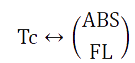Note:

Tc represents the concentration of tetracycline in the medium; ABS and FL represents the ABS value and fluorescence intensity in the bacterial fluid after 24 hours of culturing.

Those variable constitute the mapping relationship shown in the above formula.

We hope that we can get a "ruler" through the experiment, so that we can measure the ABS value and fluorescence intensity in the verification experiments, and then reversing the concentration of tetracycline in the culture medium and even in the natural environment. That is: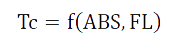Taking the E.coli strain( DH5α ) that converted into the plasmid pSB1C3 as an example: Draw our experimental data (a total of 400) into a diagram as follows, as circled in the red box, and you can see that there are some very "strange" data here, which may be the result of misoperation in some of our experiments. We call these outliers and it represents the data is needed to be cleaned.Taking the E.coli strain( DH5α ) that cnverted into the plasmid pSB1C3 as an example: Draw our experimental data (a total of 400) into a diagram as follows, as circled in the red box, and you can see that there are some very "strange" data here, which may be the result of misoperation in some of our experiments. We call these outliers and it represents the data is needed to be cleaned.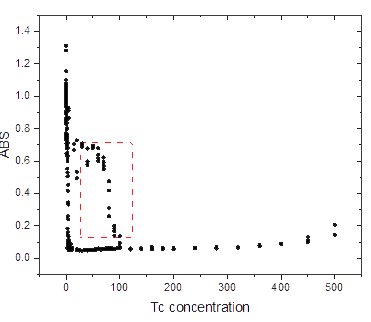### 1.1 LOF algorithm:

We use the Local Outlier Factor (LOF) to outlier mining.

Among many outlier detection methods, LOF method is a typical density-based high-precision outlier detection method. In the LOF method, each data point is assigned an outlier factor LOF which depends on the density of neighborhood to determine whether the data point is outlier. If LOF is much larger than 1, the data point is outlier; if LOF is close to 1, the data point is positive constant.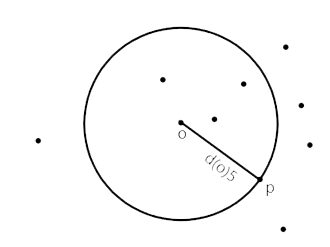The k distance is defined as the point at the fifth distance from point O (as shown above), represented by D (o) 5 or D (o, 5). The following conditions are satisfied: there are at least k points P', so that D (O, P') is less than (O, P'), and at most k-1 points in the set, so that D (O, P') is less than (O, P').

Thek-distance field is defined as the set of all points within the k-distance, represented by N (o) 5, and each point satisfies:D (O, P') < D (O, P) .

The definition of point O to point P's K reachable distance isd (O, P) k = max{d (O, K), OP} .

Define the local reachable density: the average reachable distance from all points in the K neighborhood of point O to o, even if the number of points on the K neighborhood boundary is greater than 1, the number of points in the range will be counted as K. If O and its neighbors are in the same cluster, the smaller the reachable distance is, the smaller the sum of the reachable distances is, and the greater the local reachable density is. If O is farther away from the neighboring point, then the reachable distance may take a larger value D (O, P), resulting in the larger the sum of reachable distances, the smaller the local reachable density.

Define a local outlier: The average of the ratio of the local reachable density of other points in the neighborhood of point O to the local reachable density of point O. If the ratio is closer to 1, it means that O has the same neighborhood density and O may belong to the same cluster as the neighborhood; if the ratio is less than 1, it means that O is denser than its neighborhood density and O is dense; if the ratio is greater than 1, it means that O is less dense than its neighborhood density, and O may be an outlier.

Using the Outlier Detection Toolbox MATLAB toolbox written by Goker Erdogan, we divide all the data into two parts to calculate, because the data is loose when the concentration of tetracycline is above 100 ug/ul. 5% data as validation data, the results are as follows: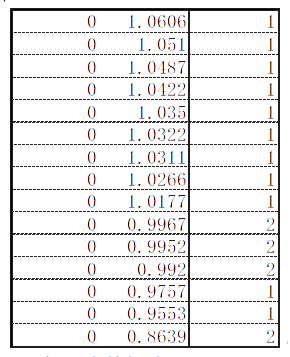1 of the data is normal and 2 indicates that the data is outlier

The abnormal data in ABS and FL are cleaned by LOF. Taking ABS data as an example, the results are as follows: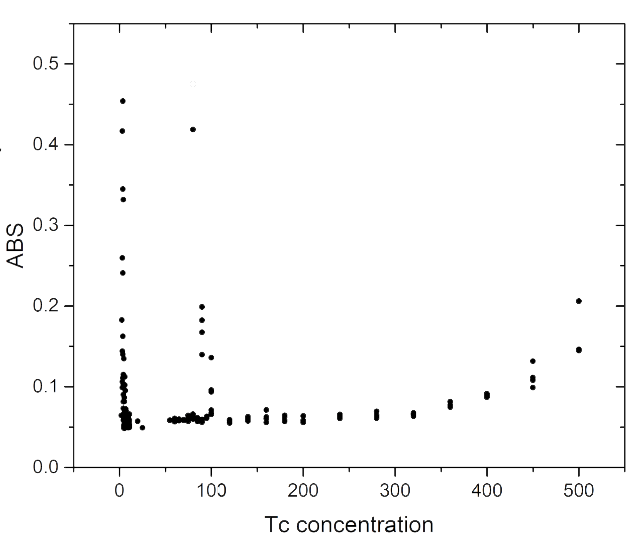### 1.2 BP neutral network：

We construct a three-layer neural network, which is input layer AI (ABS and FL), middle hidden layer Vj and output layer t. Therefore, the input layer node is 2 and the output layer is 1. The number of neurons in the middle hidden layer will greatly affect the accuracy. If it is too large, it may cause slow convergence and over-fitting problems. If it is too small, the accuracy will be reduced. Therefore, we should refer to the following empirical formula: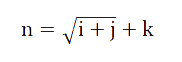Among them,

N - the number of neurons in the hidden layer;
I - the number of neurons in the input layer.
J - the number of neurons in the output layer.
K -- any number between [1,10].

According to this formula, we finally decided to make 4 our last choice.

## 2. the choice of incentive function:

BP neural network usually uses Sigmoid differentiable function and linear function as the excitation function of the network. In this paper, the S-type tangent function Tansig is chosen as the excitation function of the hidden layer neurons. It belongs to the sigmoid function and is expressed in the following form: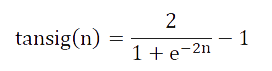The output layer excitation function is logsis function, that is: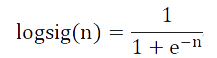The grid training function is selected as the gradient descent method with adaptive learning rate and momentum factor -- Traingdx Function.

## 3. Other Parameters Are As Follows:

After normalizing the training sample data, the network performance function is mse, and the number of hidden layer neurons is calculated by trial and error method. The number of iterations is epochs 500 times, the expected error goal is 1e-5, and the learning rate LR is 0.01.

Enter our data using the MATLAB neural network toolbox: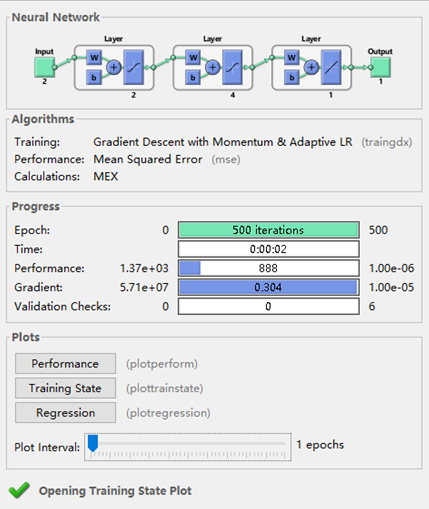It can be seen that this is an input dual neuron, output single neuron, and the number of hidden layer nodes in the middle of the neural network, running time only 2 seconds, convergence faster.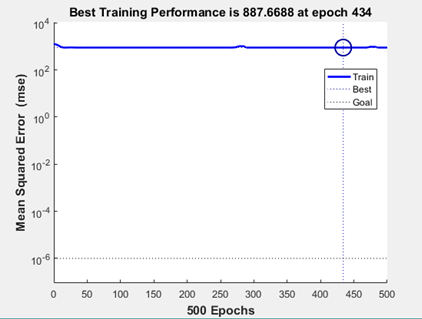After about 434 repetitions, the minimum error is reached and the learning stops, but the error is still large and the target value can not be reached.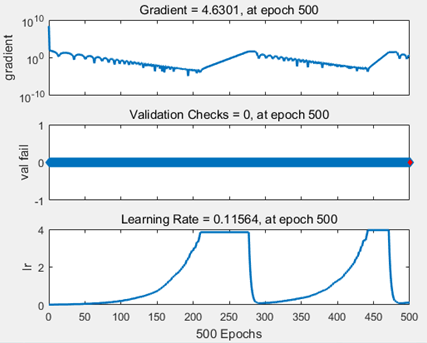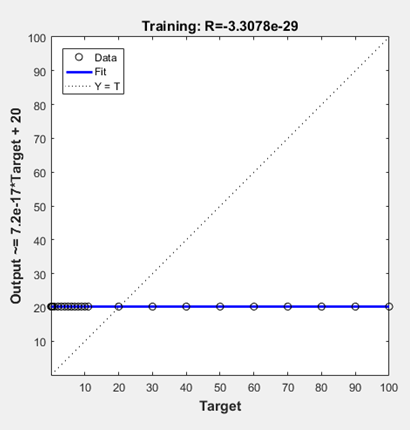## 4.Dynamics of Free Growth

In order to understand the effect of the plasmids we constructed on the detection of tetracycline, it is very important to understand the growth of microorganisms and establish a model. In recent years, the study of growth dynamics model has become the focus of predicting microbial growth. It is often divided into primary prediction model and secondary prediction model.

In the primary prediction model, the modified Monod equation, Logistic equation, Baranyi-Roberts model and Gompertz equation are common. After consulting the literatures, we decided to select Gompertz equation as the equation of this forecast temporarily. Its basic form is: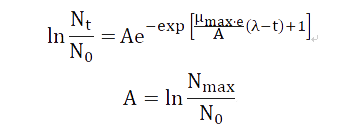Two directions for time t: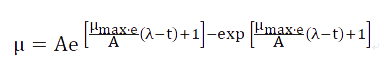N_0—Number of microbes at zero time;
N_t—Number of microbes at t time;
N_max—The maximum number of microbes;
λ—The length of the delay period.

Because the quantity of microorganism is difficult to measure and we think the volume of culture medium does not change during culture, the quantity of microorganism is proportional to the density and the density is proportional to the ABS value.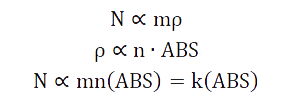Therefore, the Gompertz equation can be transformed into: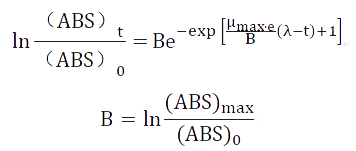Symbol Number Unite Note （ABS） Variable - Coming from measurement （ABS）0 To be measured - ABS value of initial time （ABS）max To be measured - Maximal ABS value μm To be measured - Coming from measurement λ Unavailable min It is not measurable, but it can be estimated approximately

* Due to the time constraint, we cannot measure all of data in the modeling formula, so we would measure those in the future work.

# Part2:

Why is the degradation efficiency of three enzymes degrade smaller than that of individual enzyme?

Suppose:

1. The larger the plasmid, the more difficult to be introduced into bacteria, and the number of plasmids introduced into a bacteria has the following relationship with the number of plasmid bp: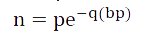P and Q are fixed values;
BP is the BP number of the plasmid.
N is the average number of plasmids introduced by each bacterium;

2. It is possible for the plasmid to be inadvertently exfoliated with ribosomes during expression. Assuming that the exfoliation probability is d for each codon, the exfoliation probability is dm for m codons.

3. the degradation efficiency varies from enzyme to enzyme, and the units are all (tetracycline /h). However, because this value is difficult to measure, we use the ABS value of the medium as a substitute for the dimensionless ABS value of the bacteria containing each enzyme after growing in the tetracycline environment for a long enough time. Supposed he degradation efficiency of lip is u, MnP is V and laccase is w. (The base number of lip is x, MNP is y, and laccase is Z.)

Taking a lip+mnp plasmid as an example, its degradation efficiency is as follows: The number of plasmid introduced into each bacterium was x (expressing the number of lip x lip degradation efficiency +The number of MNP x expressed MNP efficiency.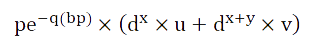The degradation efficiency of a MP + lip plasmid is: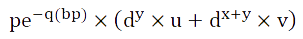We can see that the degradation efficiency of plasmid is different from that of two identical enzymes, and because the larger the plasmid is, the smaller the n is, it is likely that the degradation efficiency of three degrading enzymes is not even as good as that of a single enzyme.foot